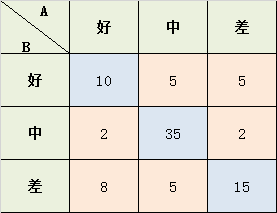# 【一致性检验指标】Kappa(cappa)系数

### 1 定义

$k = \frac{p_0-p_e}{1-p_e}$

$p_0$是每一类正确分类的样本数量之和除以总样本数，也就是总体分类精度

#### 1.1 简单例子Po = (10+35+15) / 87 = 0.689
a1 = 10+2+8 = 20; a2 = 5+35+5 = 45; a3 = 5+2+15 = 22;
b1 = 10+5+5 = 20; b2 = 2+35+2 = 39; b3 = 8+5+15 = 28;
Pe = (a1b1 + a2b2 + a3b3) / (8787) = 0.455
K = (Po-Pe) / (1-Pe) = 0.4293578

### 2 指标解释

kappa计算结果为[-1,1]，但通常kappa是落在 [0,1] 间

0.0~0.20极低的一致性(slight)

0.21~0.40一般的一致性(fair)

0.41~0.60 中等的一致性(moderate)

0.61~0.80 高度的一致性(substantial)

0.81~1几乎完全一致(almost perfect)

posted @ 2020-05-23 02:20  忽逢桃林  阅读(...)  评论(...编辑  收藏